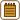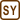## Oil Pipetting

Determine the surface tension of oil with density of 0.910 g·cm−3, if 304 oil drops were made during pipetting 4.0 cm3 of oil. Diameter of the pipette is 1.2 mm.

• #### Hint

A droplet is getting larger and it will drop at the moment when the magnitude of its weight, which is directed downwards, is greater than the magnitude of the force of the oil’s surface tension which, on the contrary, holds the droplet at the end of the pipette.

Surface tension force is directly proportional to the surface tension of oil and to the length at which it acts, i.e. to the circumference of the pipette.

• #### Notation

 ρ = 0.91 g cm−3 = 910 kg m−3 oil density d = 1.2 mm = 1.2·10−3 m diameter of the pipette V = 4.0 cm3 = 4.0·10−6 m3 total volume of oil N = 304 number of drops σ = ? desired surface tension of oil (in air)
• #### Analysis

During the pipetting, a droplet forms at the end of the pipette. The droplet is getting bigger and bigger until its weight (which pulls it downwards) is equal to the surface tension force which holds the droplet at the pipette's tip. If the drop increases in size even more, it will come off and a new droplet will start to form at the tip of the pipette.

We determine the volume of one drop from the total volume of the oil and the number of drops, and then we will easily compute the weight of one droplet. The magnitude of the surface tension force is directly proportional to the length of the edge of the liquid surface, in our case, to the circumference of the pipette and the surface tension. From the equality of these two forces we can determine the desired surface tension of oil.

• #### Solution

First, we determine the volume of one droplet V1

$V_1\,=\, \frac{V}{N}$

and using this volume, we calculate the magnitude of the weight W of one oil drop

$W\,=\,mg\,=\,V_1 \varrho g\,=\,\frac{V}{N}\varrho g.$

Next, we determine the magnitude of the surface tension force F, which acts at the circumference of the pipette c = πd

$F\,=\, \sigma \, c \,=\,\sigma \pi d$

Then we compare these two forces

$W\,=\, F$ $\frac{V}{N}\varrho g \,=\,\sigma \pi d$

and express the desired surface tension of oil

$\sigma\,=\,\frac{V\varrho g }{N\pi d} \,=\,\frac{ 4\cdot{10^{-6}}\,\cdot \,910\,\cdot \,9.81}{304\,\cdot \,\pi \,\cdot \,1.2\cdot{10^{-3}}}\,\dot=\,0.031\,\mathrm{N\,m^{-1}}$ $\sigma\,\dot=\,31\,\mathrm{mN\,m^{-1}}$